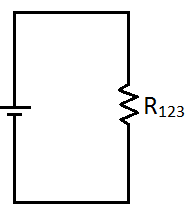# AP Physics C Electricity : Calculating Resistance

## Example Questions

### Example Question #63 : Electricity And Magnetism Exam

Three resistors and a battery form the following circuit.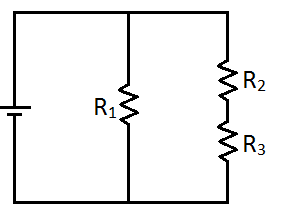Calculate the equivalent resistance if the resistors have the following values.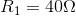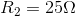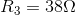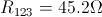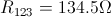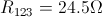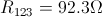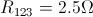Explanation:

First, calculate the equivalent resistance of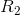and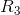. Since these two resistors are arranged in series, we just take the sum of their values.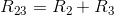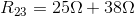With resistors 2 and 3 combined together in a single value, the following circuit is formed.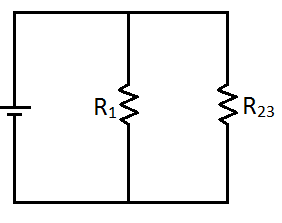Notice that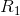and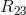are arranged in parallel. To calculate the equivalent resistance of this parallel pair, we use the following equation.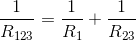Plug in the values, and solve for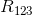.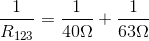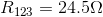This is just like the circuit shown below.